>  Ratio And Proportion, Indices And Logarithm

# Ratio And Proportion, Indices And Logarithm

Test Description

## 70 Questions MCQ Test | Ratio And Proportion, Indices And Logarithm

 1 Crore+ students have signed up on EduRev. Have you?
Ratio And Proportion, Indices And Logarithm - Question 1

### Two numbers are in the ratio 2 : 3 and the difference of their squares is 320. The numbers are : [2006-November]

Ratio And Proportion, Indices And Logarithm - Question 2

### If p : q is the sub-duplicate ratio of p - x2: q - x2, then x2 is:    [2006-November]

Ratio And Proportion, Indices And Logarithm - Question 3

### An alloy is to contain copper and zinc in the ratio 9 : 4. The zinc required to melt with 24 kg of copper is:  [2006-November]

Ratio And Proportion, Indices And Logarithm - Question 4

7 log16/5  + 5 log25/24 + 3 log81/80 is equal to:   [2006-November]

Ratio And Proportion, Indices And Logarithm - Question 5

Two numbers are in the ratio 7:8- If 3 is added to each of Them, their ratio becomes 8 : 9. The numbers are:  [2007 - February]

Ratio And Proportion, Indices And Logarithm - Question 6

A box contains Rs.56 In the form of coins of one rupee, 50 paise and 25 paise. The number of 50 paise coin is double the number of 25 paise coins and four times the numbers of one rupee coins. The numbers of 50 paise coins in the box is:   [2007 - February]

Ratio And Proportion, Indices And Logarithm - Question 7

Value of (a1/8 + a-1/8 ) (a1/8 - a-1/8 ) (a1/4 + a-1/4 ) (a1/2 + a-1/2 ) is:    [2007 - February]

Ratio And Proportion, Indices And Logarithm - Question 8

The value of the expression: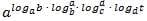[2007 - February]

Ratio And Proportion, Indices And Logarithm - Question 9

If log1000 � = -1/4  then x is given by:    [2007 - February]

Ratio And Proportion, Indices And Logarithm - Question 10

Eight people are planning to shareequally the cost of a rental car. If one person withdraws from thearrangement and the others shareequally entire cost of the car, then the share of each of the remaining persons increased by:  [2007- May]

Ratio And Proportion, Indices And Logarithm - Question 11

A bag contains Rs.187 in the form of 1 rupee, 50 paise and 1 paise coins in the ratio 3:4:5 Find the number of each type of coins :    [2007- May]

Ratio And Proportion, Indices And Logarithm - Question 12

Simplification of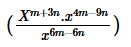is      [2007- May]

Ratio And Proportion, Indices And Logarithm - Question 13

If log (2a – 3b) = log a - log b, then a = :   [2007- May]

Ratio And Proportion, Indices And Logarithm - Question 14

On simplification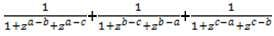reduces to :    [2007 - August]

Ratio And Proportion, Indices And Logarithm - Question 15

Ratio of earnings of A and B is 4 : 7. If the earnings of A increase by 50% and those of A increase by 25% the new ratio of their earning becomes 8 : 7 What is A’s earning ?   [2007 - August]

Ratio And Proportion, Indices And Logarithm - Question 16

P,Q and R are three cities. The ratio of average temperature between P and Q is 11 : 12 and that between P and R is 9 : 8. The ratio between the average temperatureof Q and R is :  [2007 - August]

Ratio And Proportion, Indices And Logarithm - Question 17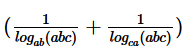is equal to :    [2007 - August]

Ratio And Proportion, Indices And Logarithm - Question 18

Number of digits in the numeral for 264 . [Given log 2 = 0.30103] :   [2007 - August]

Ratio And Proportion, Indices And Logarithm - Question 19

Rs.407 are to be divided among A, Band C so that their shares are in the ratio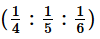.  The respective shares of A, B, C are :     [2007 - November]

Ratio And Proportion, Indices And Logarithm - Question 20

The incomes of A and B are in theratio 3 : 2 and their expenditures inthe ratio 5:3. If each saves Rs.1,500.ThenB’s income is :   [2007 - November]

Ratio And Proportion, Indices And Logarithm - Question 21

If 4x = 5y = 20z   then z is equal to :    [2007 - November]

Ratio And Proportion, Indices And Logarithm - Question 22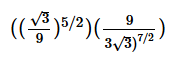� 9 is equal to     [2007 - November]

Ratio And Proportion, Indices And Logarithm - Question 23

The value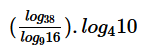is                [2007 - November]

Ratio And Proportion, Indices And Logarithm - Question 24

In 40 litres mixture of glycerine and water, the ratio ofglycerine and water is 3:1 The quantity of water added in the mixture in order to make this ratio 2:1 is :   [2008 - February]

Ratio And Proportion, Indices And Logarithm - Question 25

The third proportional between (a2 - b2) and (a + b)2  is :      [2008 - February]

Ratio And Proportion, Indices And Logarithm - Question 26

If 2x - 2x-1 = 4 then xx  is equal to :    [2008 - February]

Ratio And Proportion, Indices And Logarithm - Question 27

If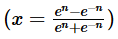then the value of n is :         [2008 - February]

Ratio And Proportion, Indices And Logarithm - Question 28

log144 is equal to :      [2008 - February]

Ratio And Proportion, Indices And Logarithm - Question 29

In what ratio should tea worth Rs.10 per kg be mixed with tea worth Rs.14per kg, so that the average price ofthe mixture may be Rs.11 per Kg?       [2008 -June]

Ratio And Proportion, Indices And Logarithm - Question 30

The ages of two persons are in theratio 5:7. Eighteen years ago theirages were in the ratio of 8:13, their present ages (in years) are :     [2008 -June]

Ratio And Proportion, Indices And Logarithm - Question 31

If x = ya , y = zb and  z = xc then abc is:    [2008 -June]

Ratio And Proportion, Indices And Logarithm - Question 32

If  log2[log3(log2x)] then x equals :   [2008 -June]

Ratio And Proportion, Indices And Logarithm - Question 33

If log (a+b)/4 = 1/2 (log a + log b) then :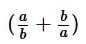[2008- December]

Ratio And Proportion, Indices And Logarithm - Question 34

If A, B and C started a business by investingRs.1,26,000, Rs.84,000 and Rs.2,10,000. If at the end of the year profit is Rs.2,42,000 then the share of each is:      [2008- December]

Ratio And Proportion, Indices And Logarithm - Question 35

If  p/q = -2/3 then the value of (2p+q)/(2p-q)  is :              [2009-June]

Ratio And Proportion, Indices And Logarithm - Question 36

Fourth proportional to x, 2x, (x+1) is:       [2009-June]

Ratio And Proportion, Indices And Logarithm - Question 37

If x = 31/3 + 3-1/3 then found the value of 3x3 - 9x      [2009-June]

Ratio And Proportion, Indices And Logarithm - Question 38

Find the value of : [1- {1-(1- x2)1/2}-1/2]        [2009-June]

Ratio And Proportion, Indices And Logarithm - Question 39

log (m + n) = log m + log n, m can be expressed as:      [2009-June]

Ratio And Proportion, Indices And Logarithm - Question 40

log4(x2 + x) - log4(x+1) = 2.Find x         [2009-June]

Ratio And Proportion, Indices And Logarithm - Question 41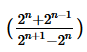[2009 - December]

Ratio And Proportion, Indices And Logarithm - Question 42

If 2x × 3y × 5z = 360  Then what is the value of x, y, z?       [2009 - December]

Ratio And Proportion, Indices And Logarithm - Question 43

Find the value of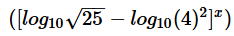[2009 - December]

Ratio And Proportion, Indices And Logarithm - Question 44

If 2x - 2x-1 = 4 then xx  is equal to :      [2009 - December]

Ratio And Proportion, Indices And Logarithm - Question 45

If loga b + loga c  = 0 then                 [2009 - December]

Ratio And Proportion, Indices And Logarithm - Question 46

What must be added to each term of the ratio 49 : 68, so that it becomes 3:4?      [2009 - December]

Ratio And Proportion, Indices And Logarithm - Question 47

The students of two classes are In the ratio 5 : 7, if 10 students left from each class, the remaining students are in the ratio of 4 : 6 then the number of students in each class is:     [2009 - December]

Ratio And Proportion, Indices And Logarithm - Question 48

The value of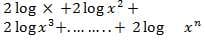will be :                            [2010 – December]

Ratio And Proportion, Indices And Logarithm - Question 49

The recurring decimal 2.7777……. can be expressed as         [2010 – December]

Ratio And Proportion, Indices And Logarithm - Question 50

Solve :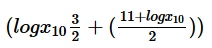[2010 – December]

Ratio And Proportion, Indices And Logarithm - Question 51

If A:B= 2:5, then (10A+3B) : (5A+2B) is equal to        [2010 – December]

Ratio And Proportion, Indices And Logarithm - Question 52

If n = m! where (‘m’ is a positive integer> 2) then the value of :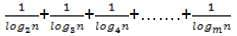[2011 – June]

Ratio And Proportion, Indices And Logarithm - Question 53

In a film shooting, A and B received money in a certain ratio and B and C also received the money in the same ratio. If A gets Rs.1,60,000 and C gets Rs.2,50,000 Find the amount received by B ?   [2011 – June]

Ratio And Proportion, Indices And Logarithm - Question 54

The ratio Compounded of 4:5 and sub-duplicate of “a”:9 is 8:15. Then Value of “a” is  [2011 – December]

Ratio And Proportion, Indices And Logarithm - Question 55

If log2x + log4x = 6, then the Value of x is                                    [2011 – December]

Ratio And Proportion, Indices And Logarithm - Question 56

If X Varies inversely as square of Y and given that Y =2 for X =1, then the Value of x for Y =6 will be    [2011 – December]

Ratio And Proportion, Indices And Logarithm - Question 57

The value of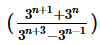is equal to         [2012 – June]

Ratio And Proportion, Indices And Logarithm - Question 58

If log y= 100 and log2x = 10, then the value of ‘y’ is:   [2012 – June]

Ratio And Proportion, Indices And Logarithm - Question 59

Which of the numbers are not in proportion ?    [2012 – June]

Ratio And Proportion, Indices And Logarithm - Question 60

Find the value of x, if x (x)1/3 = (x1/3)x         [2012 – December]

Ratio And Proportion, Indices And Logarithm - Question 61

Which of the foll  owing is true.

If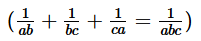[2012 – December]

Ratio And Proportion, Indices And Logarithm - Question 62

Find two numbers such that mean proportional between them is 18 and third proportional between them is 144    [2012 – December]

Ratio And Proportion, Indices And Logarithm - Question 63

For what value of x, the equation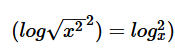is true?      [2013 – June]

Ratio And Proportion, Indices And Logarithm - Question 64

The mean proportional between 24 and 54 is:     [2013 – June]

Ratio And Proportion, Indices And Logarithm - Question 65

The triplicate ratio of 4 : 5 is:        [2013 – June]

Ratio And Proportion, Indices And Logarithm - Question 66

If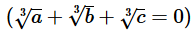, then the value of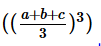[2013 – December]

Ratio And Proportion, Indices And Logarithm - Question 67

Find three numbers in the ratio 1 : 2 :3, so thatthe sum of their squares is equal to 504     [2013 – December]

Ratio And Proportion, Indices And Logarithm - Question 68

The value of log49 . log32 is          [2013 – December]

Ratio And Proportion, Indices And Logarithm - Question 69

The value of (logyx . logzy . logxz)3 is        [2013 – December]

Ratio And Proportion, Indices And Logarithm - Question 70

Divide 80 into two parts 50 that their product is maximum, then the numbers are     [2013 – December]

 Use Code STAYHOME200 and get INR 200 additional OFF Use Coupon Code